# In-Class Acitivity: Analysis of Variance (ANOVA)

We are going to use R.appendix1.data from Personality Project. We will load and print the data.

# 1. Loading
data1
   Dosage Alertness
1       a        30
2       a        38
3       a        35
4       a        41
5       a        27
6       a        24
7       b        32
8       b        26
9       b        31
10      b        29
11      b        27
12      b        35
13      b        21
14      b        25
15      c        17
16      c        21
17      c        20
18      c        19
# 2. Print
head(data1)
  Dosage Alertness
1      a        30
2      a        38
3      a        35
4      a        41
5      a        27
6      a        24

## Check Data Set

Check the number of rows and columns.

# Check the dimension of an object
dim(data1)
 18  2
# Display the internal structure of an R object.
str(data1)
'data.frame':   18 obs. of  2 variables:
$Dosage : Factor w/ 3 levels "a","b","c": 1 1 1 1 1 1 2 2 2 2 ...$ Alertness: int  30 38 35 41 27 24 32 26 31 29 ...
# Check the levels
levels(data1$Dosage)  "a" "b" "c" ## Visualize your data - Boxplot & Histogram # par(mar=c(1,1,1,1)) # change figure margins if you encounter error # Boxplot boxplot(data1$Alertness)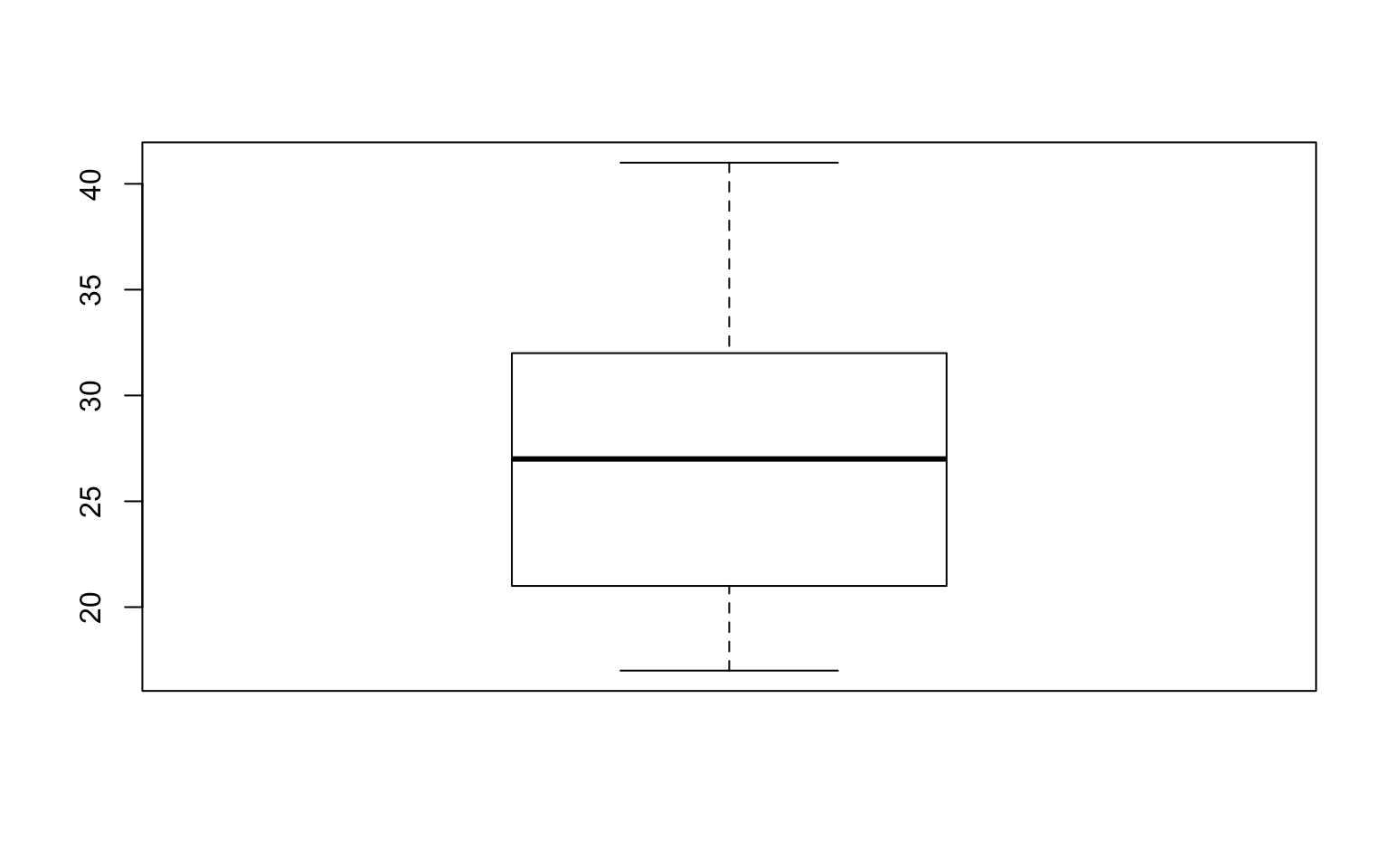# Boxplot (Alterness by group)
boxplot(data1$Alertness ~ data1$Dosage)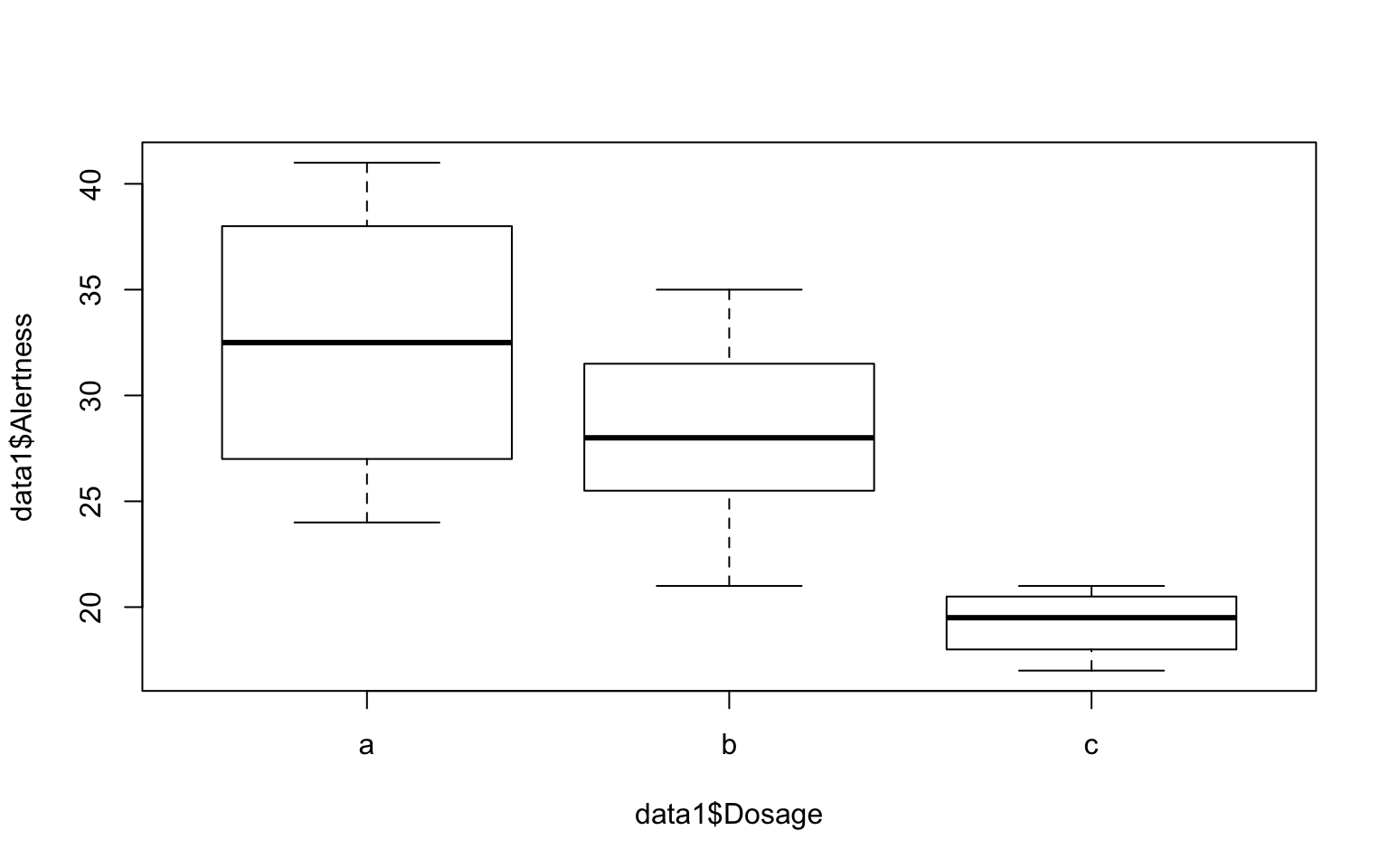# Histogram
qqline(cars$dist)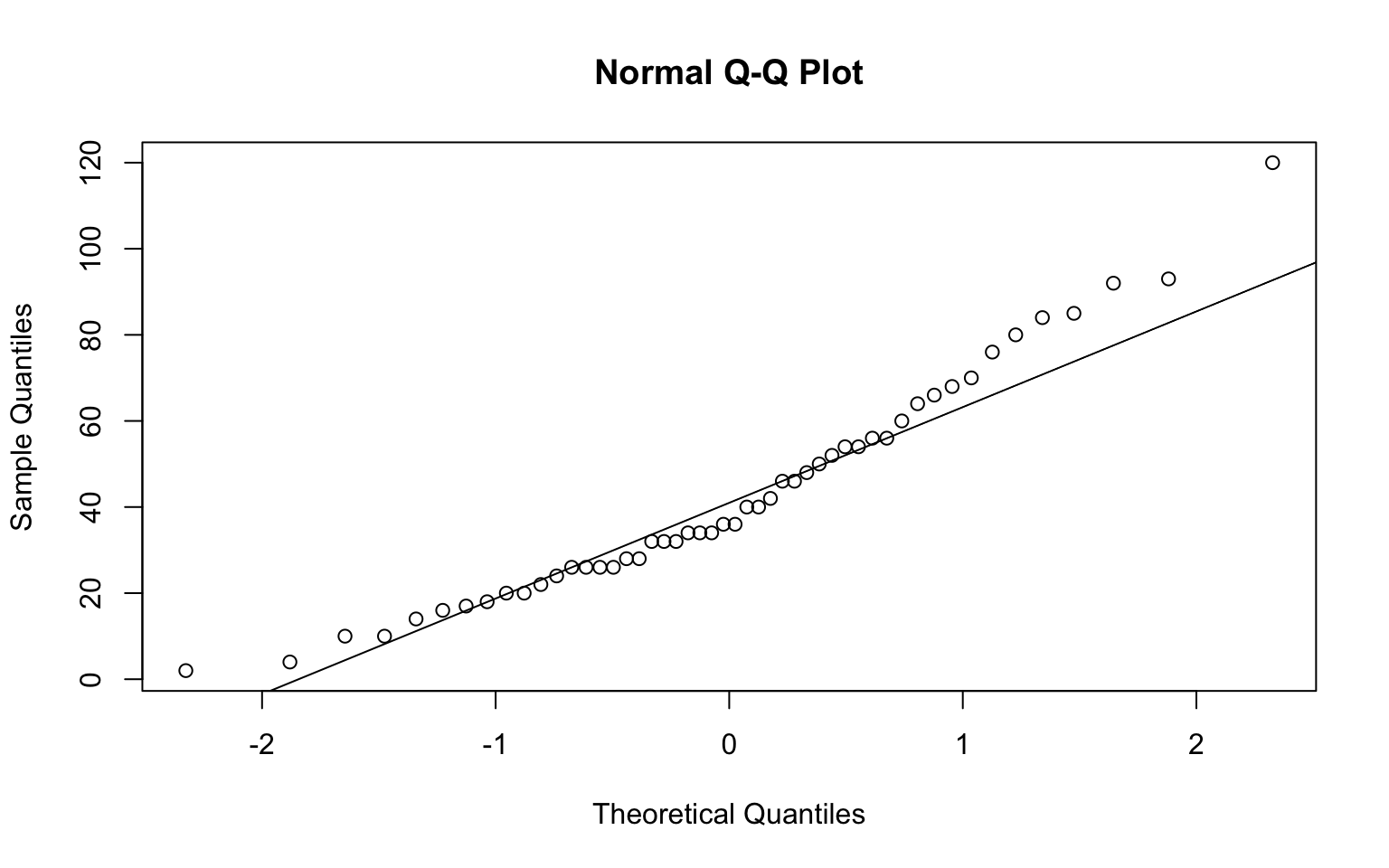# Use plot function to get a QQ plot plot(aov.ex1, 2)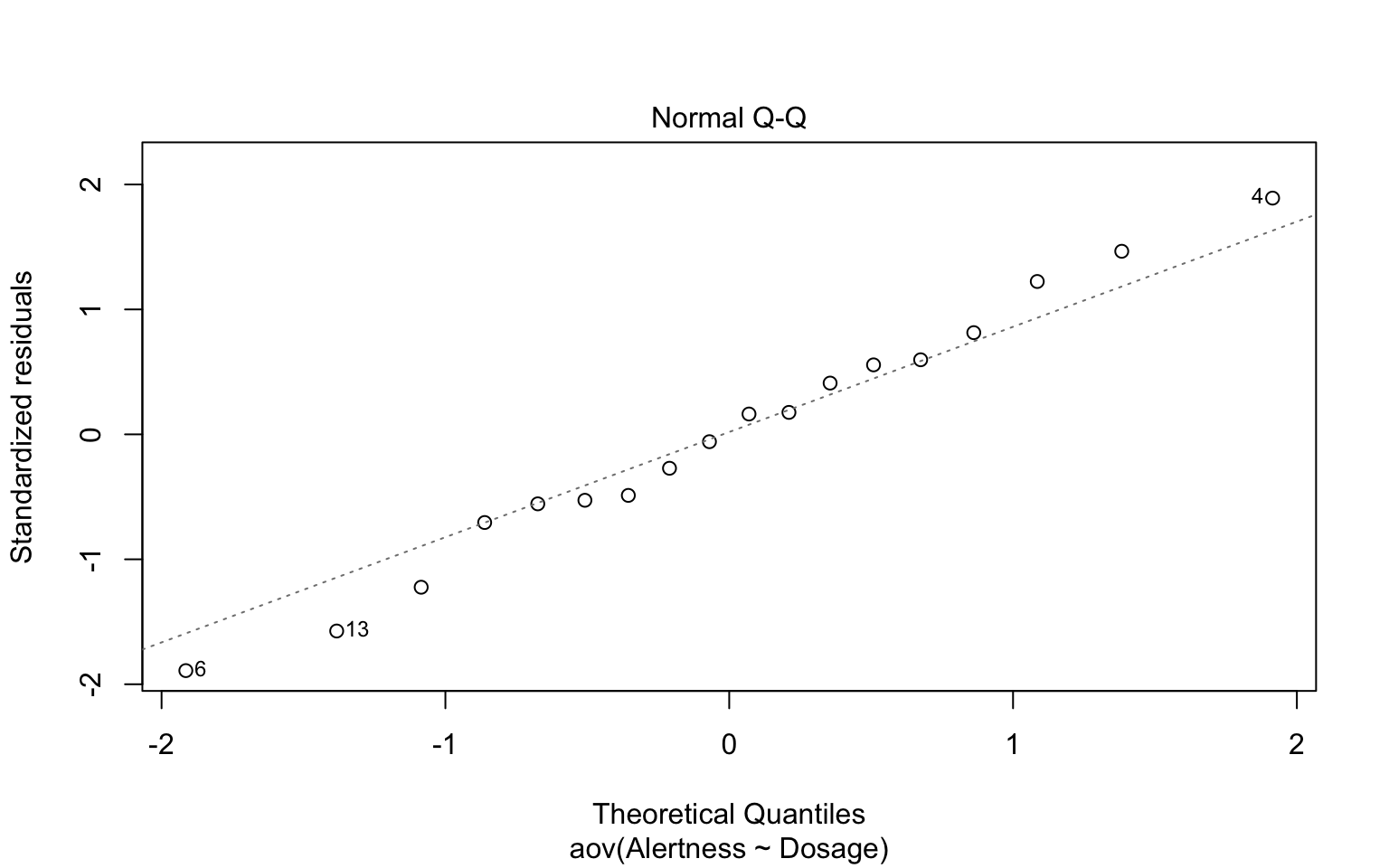# Run Shapiro-Wilk test shapiro.test(x = residuals(aov.ex1))  Shapiro-Wilk normality test data: residuals(aov.ex1) W = 0.98604, p-value = 0.991 ## Non-parametric alternative to one-way ANOVA test: Kruskal-Wallis rank sum test When ANOVA assumptions are not met, you cna use a non-parametric alternative. kruskal.test(Alertness ~ Dosage, data = data1)  Kruskal-Wallis rank sum test data: Alertness by Dosage Kruskal-Wallis chi-squared = 9.4272, df = 2, p-value = 0.008972 # In-Class Acitivity: Multiple pairwise-comparison ## Bonferroni and Holm multiple pairwise-comparisons In one-way Anova, a siginificant p-value means some of the group means are different. However, this does not tell us which pairs of groups are different. We can perform multiple pairwise-comparison to test the statistically significant mean difference between particular pairs of group. Given the significant ANOVA test, we can compute adjusted p-values using pairwise.t.test. # no adjustment pairwise.t.test(data1$Alertness, data1$Dosage, data = , p.adj = "none")  Pairwise comparisons using t tests with pooled SD data: data1$Alertness and data1$Dosage a b b 0.13088 - c 0.00082 0.00926 P value adjustment method: none  # Bonferroni pairwise.t.test(data1$Alertness, data1$Dosage, data = , p.adj = "bonf")  Pairwise comparisons using t tests with pooled SD data: data1$Alertness and data1$Dosage a b b 0.3926 - c 0.0025 0.0278 P value adjustment method: bonferroni  # Holm pairwise.t.test(data1$Alertness, data1$Dosage, data = , p.adj = "holm")  Pairwise comparisons using t tests with pooled SD data: data1$Alertness and data1$Dosage a b b 0.1309 - c 0.0025 0.0185 P value adjustment method: holm  ## Tukey multiple pairwise-comparisons Tukey HSD (Tukey Honest Significant Differences) using TukeyHD. # diff: difference between means of the two groups lwr, upr: the lower and # the upper end point of the confidence interval at 95% (default) p adj: # p-value after adjustment for the multiple comparisons. TukeyHSD(aov.ex1)  Tukey multiple comparisons of means 95% family-wise confidence level Fit: aov(formula = Alertness ~ Dosage, data = data1)$Dosage
b-a  -4.25 -11.15796  2.657961 0.2768132
c-a -13.25 -21.50659 -4.993408 0.0022342
c-b  -9.00 -16.83289 -1.167109 0.0237003

# In-Class Acitivity: Planned Constrasts

You can test some specific hypotheses than testing all possible mean comparisons. We can combine multiple means from different levels and compare two means (e.g., a and b vs. c)

# assign values to the groups that you want to compare
c1 <- c(0.5, -0.5, 0)  # H0_c1: a = b
c2 <- c(0.5, 0.5, -1)  # H0_c2: a & b = c

# assign the contrasts
data1$c1 <- c(rep(0.5, 6), rep(-0.5, 8), rep(0, 4)) data1$c2 <- c(rep(0.5, 6), rep(0.5, 8), rep(-1, 4))

# compare differences between pairs of means (using regression)
anova(lm(Alertness ~ c1 + c2, data = data1))
Analysis of Variance Table

Df Sum Sq Mean Sq F value   Pr(>F)
c1         1  42.98   42.98  1.7722 0.202990
c2         1 383.27  383.27 15.8051 0.001218 **
Residuals 15 363.75   24.25
---
Signif. codes:  0 '***' 0.001 '**' 0.01 '*' 0.05 '.' 0.1 ' ' 1

# FYI: Visualize your data using ggpubr

## Bar plots with mean +/- se with jittered points

# Install R package ggpubr

## Recommended: Install the latest developmental version from GitHub (remove
## # below) if(!require(devtools)) install.packages('devtools')
## devtools::install_github('kassambara/ggpubr')

## If that failed, try one from CRAN (Remove # below)
## install.packages('ggpubr') install.packages('ggplot2')

library(ggpubr)
library(ggplot2)

ggbarplot(data1, x = "Dosage", y = "Alertness", add = c("mean_se", "jitter"))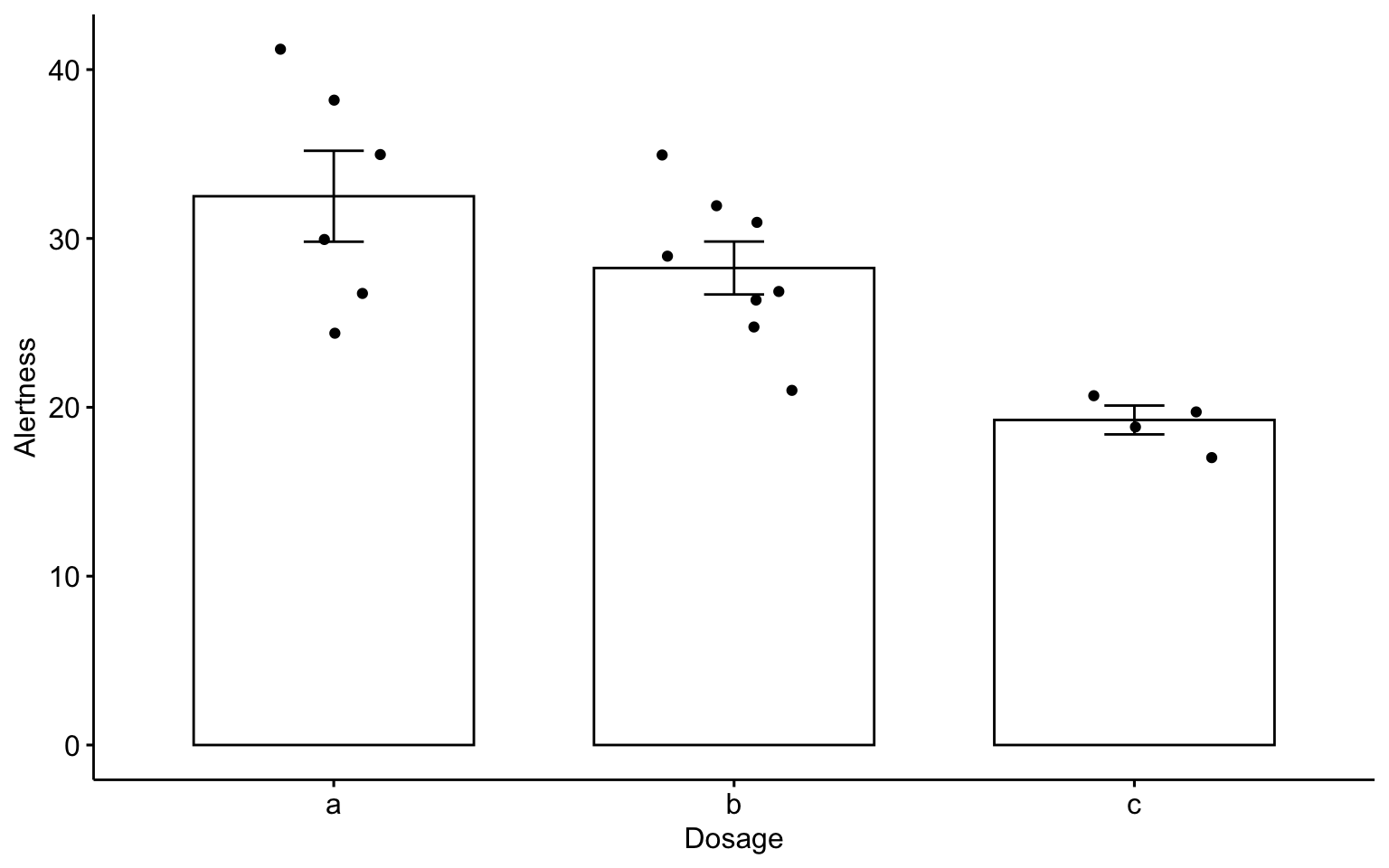# In-Class Acitivity: Tidyverse

tidyverse is an opinionated collection of R packages designed for data science. https://www.tidyverse.org/ This is designed to make it easy to install and load core packages from the tidyverse. magrittr offers a set of operators which make your code more readable such as the pipe operator %>% https://magrittr.tidyverse.org/ dplyr provides a set of tools for efficiently manipulating datasets: https://dplyr.tidyverse.org/.

# The easiest way to get dplyr or magrittr is to install the whole
# tidyverse: install.packages('tidyverse')

# Alternatively, install just dplyr or magritter: install.packages('dplyr')
# install.packages('magrittr')

library(magrittr)
library(dplyr)
library(tidyverse)

## Computes summary tables using dplyr

data1 %>% group_by(Dosage) %>% summarise(count = n(), mean = mean(Alertness,
na.rm = TRUE), sd = sd(Alertness, na.rm = TRUE))
# A tibble: 3 x 4
Dosage count  mean    sd
<fct>  <int> <dbl> <dbl>
1 a          6  32.5  6.60
2 b          8  28.2  4.43
3 c          4  19.2  1.71

# Assignment 10 (Week 15)

We will use build-in data set Prestige contained in the R package car. We will load and print the survey data.

# 1. Load the required package. install.packages('car') # if you don't have
# the 'car' package, make sure to install it.
library(car)

data(Prestige)

# 2. Print
head(Prestige)
                    education income women prestige census type
gov.administrators      13.11  12351 11.16     68.8   1113 prof
general.managers        12.26  25879  4.02     69.1   1130 prof
accountants             12.77   9271 15.70     63.4   1171 prof
purchasing.officers     11.42   8865  9.11     56.8   1175 prof
chemists                14.62   8403 11.68     73.5   2111 prof
physicists              15.64  11030  5.13     77.6   2113 prof

## Check Data

Check the number of rows and columns.

# Display the internal structure of an R object.
str(Prestige)
'data.frame':   102 obs. of  6 variables:
$education: num 13.1 12.3 12.8 11.4 14.6 ...$ income   : int  12351 25879 9271 8865 8403 11030 8258 14163 11377 11023 ...
$women : num 11.16 4.02 15.7 9.11 11.68 ...$ prestige : num  68.8 69.1 63.4 56.8 73.5 77.6 72.6 78.1 73.1 68.8 ...
$census : int 1113 1130 1171 1175 2111 2113 2133 2141 2143 2153 ...$ type     : Factor w/ 3 levels "bc","prof","wc": 2 2 2 2 2 2 2 2 2 2 ...
# Check the dimension of an object
dim(Prestige)
 102   6

## Use the help function to learn about variables

# A question mark is a shorcut for the 'help' function.
?(Prestige)